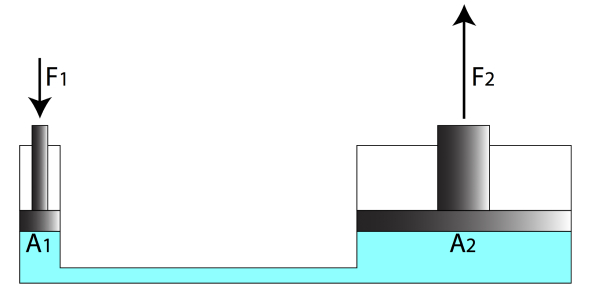# Pascal's Principle Quiz

10 Questions | Total Attempts: 4318SettingsCreate your own QuizAre you aware of Pascal's principle? Take this informative " Pascal's Principle Quiz" to test your knowledge. Pascal’s principle, also called Pascal’s law, in fluid (gas or liquid) mechanics, statement that in a fluid at rest in a closed container, a pressure change in one part is transmitted without loss to every portion of the fluid and to the walls of the container. If you have a physics test then it's the perfect quiz to test your basics. Good Luck! .

• 1.
Which of the following is true about the hydraulic system?
• A.

The pressure exerted on a smaller piston is greater than on the larger piston.

• B.

The pressure exerted on a larger piston is greater than on the smaller piston.

• C.

The pressure exerted on a small piston is equal to that on the larger piston.

• D.

No statement is true

• 2.
A barber raises his customer’s chair by applying a force of 150N to a hydraulic piston of area 0.01 m2. If the chair is attached to a piston of area 0.1 m2, how massive a customer can the chair raise? Assume the chair itself has a mass of 5 kg.
• A.

148 kg

• B.

130 kg

• C.

1500 kg

• D.

200 Kg

• 3.
A hydraulic system is used to lift a 2000 kg vehicle in an auto garage. If the vehicle sits on a piston of area 0.5 square meter, and a force is applied to a piston of area 0.03 square meters, what is the minimum force that must be applied to lift the vehicle?
• A.

120 N

• B.

550 N

• C.

1176 N

• D.

1200N

• 4.
Which of the following is the application of hydraulic system?
• A.

Heat engines and cardiovascular system

• B.

Heat engines and heat pumps

• C.

Cardiovascular system and jacks

• D.

Thermal engines and electrical engines

• 5.
Which of the following statements is true?
• A.

If the second area is greater than the first area, then the second force exerted on the system should be greater than the first force.

• B.

Pascal principle states that the pressure exerted to a confined fluid is not transmitted uniformly in all direction.

• C.

Pressure exerted on the system is directly proportional to the volume.

• D.

Pressure exerted on the system is inversely proportional to the volume.

• 6.
Does pascal's law apply to gases as well?
• A.

True

• B.

False

• C.

I don't know

• D.

Maybe

• 7.
Which is the practical application of the Pascal's law?
• A.

Hydraulic Press

• B.

Hydraulic Jack System

• C.

Brake System

• D.

All Of The Above

• 8.
When was Pascal's Law discovered?
• A.

1652

• B.

1653

• C.

1654

• D.

1655

• 9.
The pressure at the bottom of the tank that has liquid doesn't depend on what?
• A.

Nature of the liquid

• B.

Acceleration due to gravity

• C.

Area of the bottom

• D.

Height of the column

• 10.
According to Archimedes' Law,  the loss of weight in the body in the liquid is equal to.
• A.

Weight of the total liquid

• B.

Weight of the body

• C.

Height of the column

• D.

Weight of liquid displaced

Related TopicsBack to top
×

Wait!
Here's an interesting quiz for you.